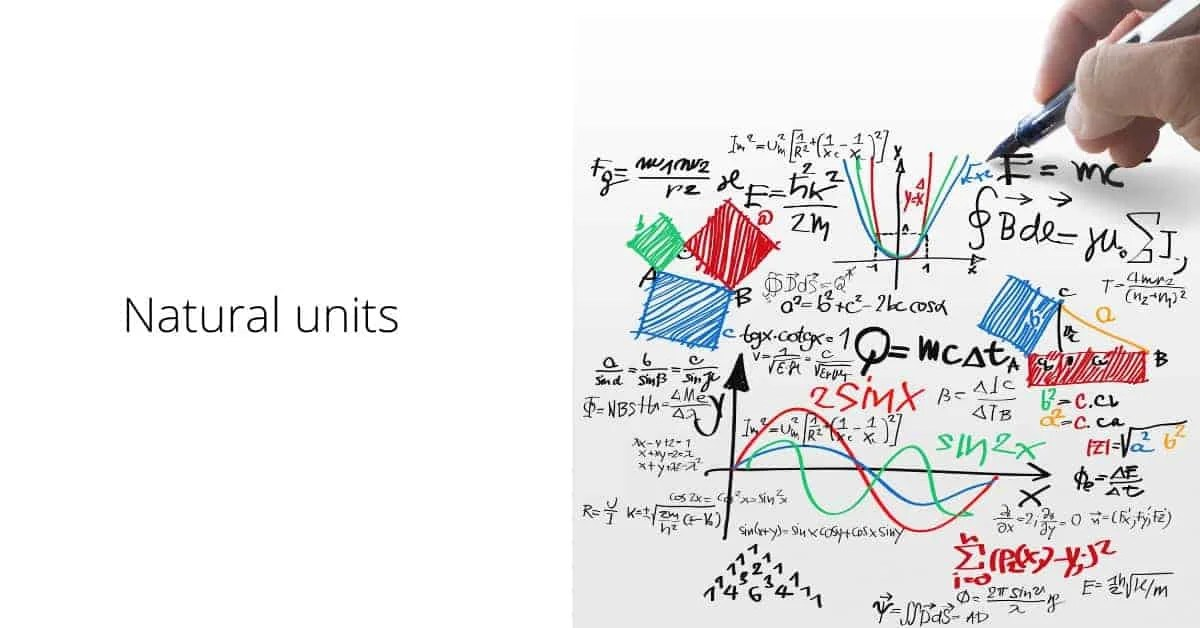# 3 Natural units and equationsParticle physicists have a habit that on the face of it seems to violate physics. They set the values of natural constants such as the speed of light and Planck’s constant to the number one. How can they do this? It’s because the relationship between units by which we measure length, time and energy is a matter of choice.

For example, we know what a second is, and we know what a meter is. But how many meters make a second? Is that like asking how many apples are there in an orange? Maybe. But not quite, because the constants of Nature like Planck’s constant and the speed of light represent natural relationships between different units of measurement, as explained below.

Space at the Speed of Light: The History of 14 Billion Years for People Short on Time#### Speed of light

The measured value of the speed of light is

so why would physicists want to pretend that instead c=1? What they are really doing is choosing a relationship between a unit of time, the second, and a unit of space, the meter, so that these two units are not independent but related. The natural constant of relation is the speed of light, so that

If we relate meters and seconds so that one second is equal to 300 million meters, then c=1. It’s very simple. Now notice that in this system of unit, mass and energy have the same units, because the relationship E = m c2 in units with c=1 just reduces to E = m.

#### Planck’s constant

Planck’s constant has units of energy x time. The preferred unit of energy in physics is the electron volt (or eV for short) is the amount of work necessary to move one electron across a potential of one volt. For particle physicists, this is a very handy unit because particle experiments use electrical potential to accelerate and bang electrons and other particles with the same charge into each other. In unit with c=1, the mass of the electron is 0.5 MeV. Written in terms of eV, Planck’s constant takes the value

The version of Planck’s constant that physicists normally use is called “h-bar” and is equal to

In c=1 units, time is described in meters, the value of Planck’s constant becomes

But physicists aren’t happy to stop there. We like to make all calculations as simple as possible. So the next step is set (this version of) Planck’s constant equal to one to set the relationship between energy units and length units so that

The size of an atom is roughly 10-10 meters. Atomic physicists use a unit called the Ångstrom, where 1 Å = 10-10 meters. Written in these terms we get the relationship

Notice that in these new units, increasing energy means decreasing length. Distances scales that are much smaller than the size of an atom have mass scales associated with them that are much larger than 2000 eV.

That is typical behavior for quantum mechanics. The de Broglie relation for wave-particle duality

also shows that in quantum physics, it is necessary to use a large energy or momentum scale to probe a small distance scale. That’s why particle accelerators are like microscopes. When the particle accelerator energy gets bigger, the distance scale being probed gets smaller.

#### Gravity

Can we play the same game with Newton’s gravitational constant? Not really, because there aren’t any new units to relate that aren’t already related. If we consider spacetimes with gravity in higher dimensions, Newton’s constant has units that depend on the dimension of spacetime. The value currently measured is, of course, for d=4.

In natural units, Newton’s constant has units of Ld-2, or M2-d.

Thus one can express Newton’s constant in units of time, length or mass, as desired.

The Planck length is thought to be the natural distance scale at which quantum gravitational effects become strong enough to notice.

Black Holes, Tides, and Curved Spacetime: Understanding GravityWatch video on Amazon- https://amzn.to/35XwWC0